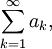# Power Series Example

Previous: The Power Series

Next: Power Series Convergence

## Example

Consider the infinite seriesDetermine the domains of these two series

## Complete Solution

### Part a)

The ratio test gives us:This limit yields infinity unless x = 0. Therefore, the domain is the single point, x = 0.

### Part b)

The ratio test gives us:This limit is zero for all real values of x. Therefore, the domain is the set of all real numbers.

## Explanation of Each Step

Because the procedure was the same for both parts a) and b), we can consider the parts together.

For both parts, we used the ratio test. Recall that the ratio test is given by the following.

The Ratio Test
To apply the ratio test to a given infinite serieswe evaluate the limitThere are three possibilities:

• if L < 1, then the series converges
• if L > 1, then the series diverges
• if L = 1, then the test is inconclusive

In part a) we found that for nonzero x, our limit was infinite, and was equal to zero when x equals zero. In part b), we found that the limit was zero, so the series converged for all x. Theses results give us the required domains of the series.

## Possible Challenges

### What Convergence Test Should Be Used?

For most problems, the ratio test can be used initially. If the ratio test yields an interval for the domain, we need to use other convergence tests to explore what the domain could be at the end points of the interval.

Previous: The Power Series

Next: Power Series Convergence